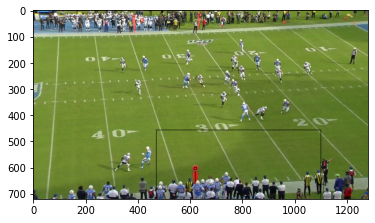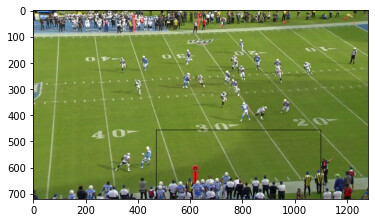# TypeError: argument must be sequence

img = Image.open("/content/drive/MyDrive//58125_000893_Sideline_frame298.jpg").convert(‘RGB’)

convert_tensor = torchvision.transforms.ToTensor()

img=convert_tensor(img)

left=1099

width=16

top=456

height=15

boxs=[top,left,width,height]

boxs=torch.tensor(boxs,dtype=torch.int)

n=tens.permute(0,1,2)

print(n.shape)

torchvision.utils.draw_bounding_boxes(image=tens.permute(0,1,2),boxes=boxs,width=2)

I have typeerror: argument must be the sequence. I try to draw a bounding box but I get an error. can anyone help to resolve this problem

`boxes` should be a tensor in the shape `[N, 4]`, so you might need to use `boxes=boxs.unsqueeze(0)`.

I resolve that problem but I stuck with another problem.

``````img = Image.open("/content/drive/MyDrive/58125_000893_Sideline_frame298.jpg").convert('RGB')
convert_tensor = torchvision.transforms.ToTensor()

img=convert_tensor(img)
width=800
height=500
top=100
left=100
boxs=[left,top,width,height]
boxs=torch.tensor([boxs],dtype=torch.uint8)
a=torch.tensor(img,dtype=torch.uint8)

a=torchvision.utils.draw_bounding_boxes(image=a,boxes=boxs,width=5)
a=a.permute(2,1,0)
a=torch.tensor(a,dtype=torch.float64)

plt.imshow(a)
plt.show()
``````

This is my code. But i get blacked image.I have a bounding box values in left,top,height,and width. So can you tell me correct sequence to draw bounding box? and the actual image is in landscape mode. But I got portrait image. Bounding box does not occur when I use transform.rotate.

Check the min. and max. values of `a` and make sure `matplotlib` can properly visualize these values without clipping. If clipping is used, you should get a warning explaining why it’s done.

I didn’t clip the tensor.
torchvision.utils.draw_bounding_boxes is not accept float32. This only accepts uint8.

``````Tensor uint8 expected, got torch.float32
``````

I get this error If I leave tensor type as empty.
So i change the tensor type to unint8 so I am getting blacked image. How to resolve this.

You would have to transform the floating point image containing values in `[0, 1]` to `[0, 255]` e.g. by multiplying it with `255.` and then transform it to `uint8`.

Fixed thank You. But the Bounding box is not fit well. [left,top,width,height]. Is this order is correct.

No, from the docs:

boxes (Tensor) – Tensor of size (N, 4) containing bounding boxes in (xmin, ymin, xmax, ymax) format. Note that the boxes are absolute coordinates with respect to the image. In other words: 0 <= xmin < xmax < W and 0 <= ymin < ymax < H.

``````img = Image.open("/content/drive/MyDrive/58125_000893_Sideline_frame298.jpg").convert('RGB')
convert_tensor = torchvision.transforms.ToTensor()

img=convert_tensor(img)
width=16
left=1099
height=15
top=456
img=img*255
boxs=[top,left,top+width,left+height]
boxs=torch.tensor(boxs,dtype=torch.int)
print(boxs)
a=torch.tensor(img,dtype=torch.uint8)
a=torchvision.utils.draw_bounding_boxes(image=a,boxes=boxs.unsqueeze(0),width=2,colors=(0,0,255))
a=a.permute(1,2,0)
plt.imshow(a)
plt.show()
``````

The bounding box is not shown. Even i try with the black image but the bounding box is not shown.

It works for me:

``````img = torchvision.transforms.ToPILImage()(torch.randn(3, 2000, 2000))
convert_tensor = torchvision.transforms.ToTensor()
img = convert_tensor(img)

width = 16
left = 1099
height = 15
top = 456
img = img*255
boxs = [top,left,top+width,left+height]
boxs = torch.tensor(boxs,dtype=torch.int)
print(boxs)
a = img.to(torch.uint8)
a = torchvision.utils.draw_bounding_boxes(image=a,boxes=boxs.unsqueeze(0),width=10)
a = a.permute(1,2,0)
plt.imshow(a)
``````

Maybe increase the `width` or the bounding box itself, since it’s tiny (16x15 pixels) compared to the expected image shape.

the bounding box is not drawn correctly what I expect. Can you explain how to use?

``````width=15
top=456
height=16
left=1099
img=img*255
boxs=[left,top,top+width,left+height]
``````

Box values are correct, I get from the CSV file.i expect a drawn bounding box in player’s helmet.

I don’t think the drawn bounding box is created using the code snippet, is it? In your code the box would be `15x16` pixels, while the currently shown one is `~600x300`?

No that bounding box argument return that bounding box. Here is my code and output.

``````img = Image.open("/content/drive/MyDrive/58125_000893_Sideline_frame298.jpg").convert('RGB')
convert_tensor = torchvision.transforms.ToTensor()

img=convert_tensor(img)
width=15
top=456
height=16
left=1099
img=img*255
boxs=[left,top,top+width,left+height]
boxs=torch.tensor(boxs,dtype=torch.int)
a=torch.tensor(img,dtype=torch.uint8)
a=torchvision.utils.draw_bounding_boxes(image=a,boxes=boxs.unsqueeze(0),width=2,colors=(0,0,255))
a=a.permute(1,2,0)
plt.imshow(a)
plt.show()
``````